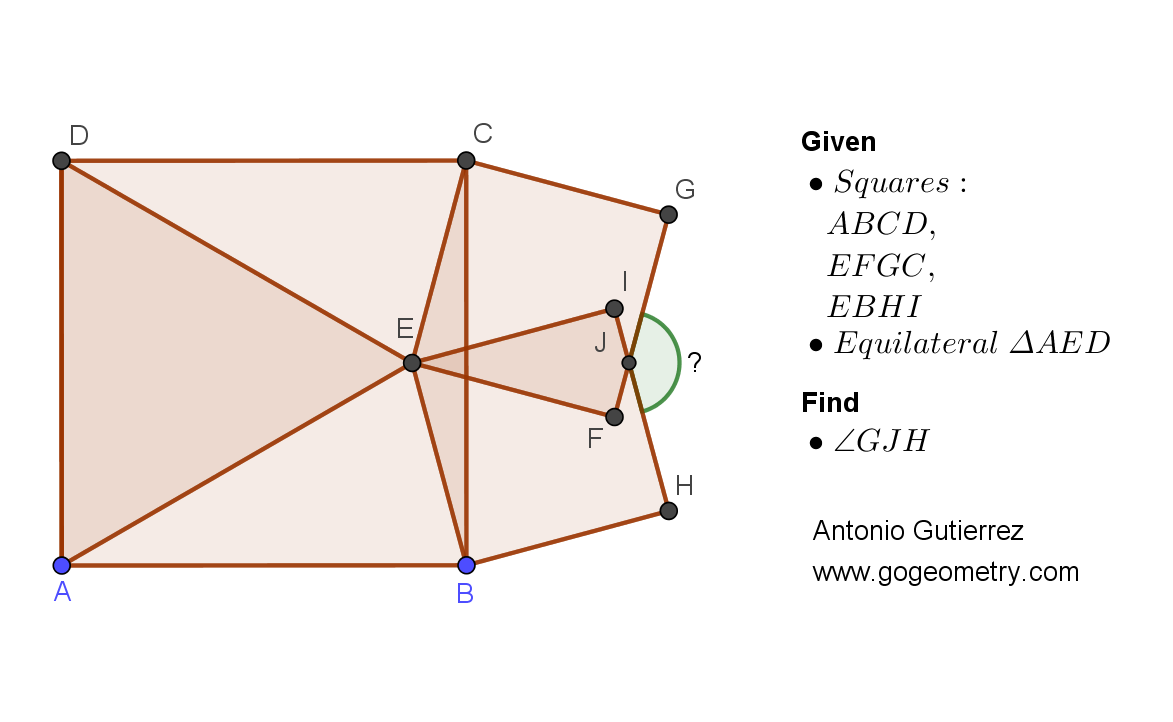## Sunday, February 12, 2023

### Geometry Problem 1504: Squares, Equilateral Triangle, Parallel, Angle

Geometry Problem 1504. Post your solution in the comment box below.
Level: Mathematics Education, K-12 School, Honors Geometry, College.

Details: Click on the figure below.More Details

To post a solution to this problem click Comment underneath the post, or click into the line that says, “Enter Comment.” Type what you want to say and press Publish to post your solution.

1.Tr. CEB is isoceles with angles 15,15,150
Tr.s CEI & BEF are congruent SAS
If < CEI = < BEF = p then considering the angles in Tr. BEC, 15+15+p + p + 90-p = 180
So p = 60 and < IEF = 30 and so < GJH = 150

Sumith Peiris
Moratuwa
Sri Lanka

1.2 interesting results

1 - FC is perpendicular to AC
2 - Tr. BIF and Tr. JFH have angles 15,30 and 135

2.A simpler solution

Since < BEC = 150 and < FEC = 90, < BEF = 60 so < EFI = 30

Hence considering cyclic quadrilateral EIJF, < GJH = 150

Sumith Peiris
Moratuwa
Sri Lanka

3.https://photos.app.goo.gl/poMiXSFHd2rRzTxS6

Construct equilateral triangle CBP as shown
Note that triangle CBP is the translation of triangle DAE with EP=DC and DE=CP
Since EP is an angle bisector of ∠(CPB) > ∠(CPE)=30
Since EP=CP -> CEP is isoceles - > ∠(CEP)=∠(ECP)= 75 and ∠(ECB)= 15
So ∠(CEB)= ∠(GJH)= 150